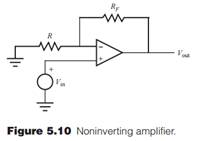Create an Account

Home / Questions / If resistor RF shown in Figure 510 is replaced with a short ie RF 0 what is the gain of the circuit

If resistor RF shown in Figure 510 is replaced with a short ie RF 0 what is the gain of the circuit

If resistor RF shown in Figure 5.10 is replaced with a short (i.e., RF = 0), what is the gain of the circuit?Jul 27 2020 View more View LessSubscribe To Get Solution